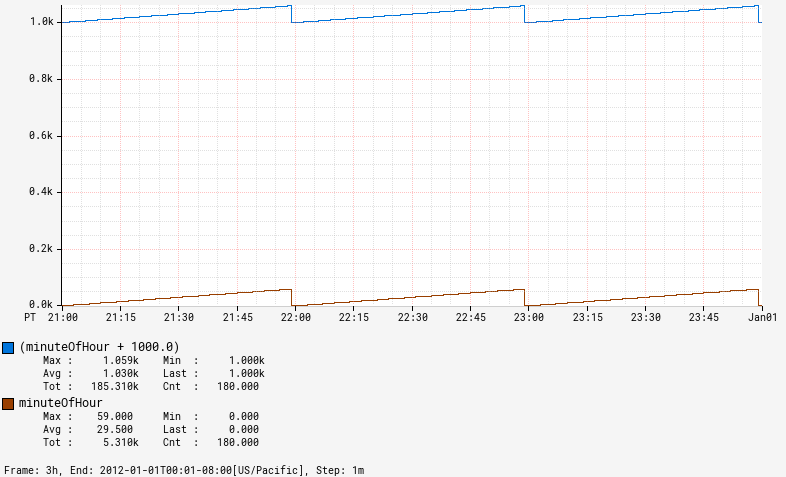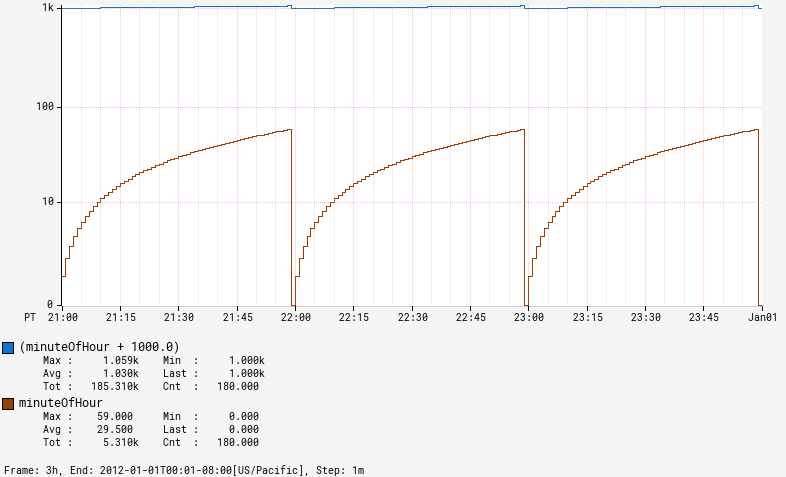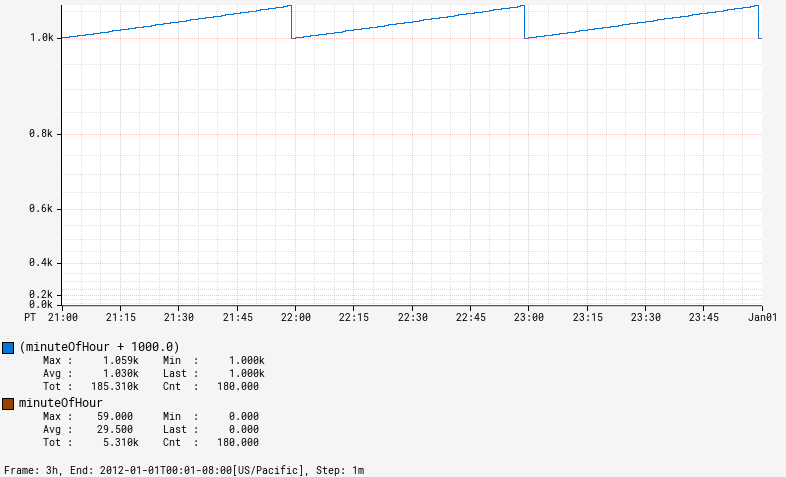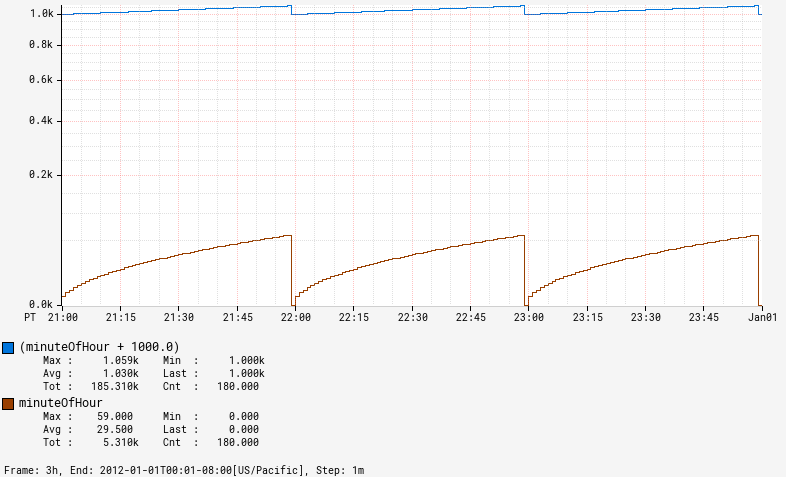# Axis Scale

Scales determine how the data value for a line will get mapped to the Y-Axis. There are currently four scales that can be used for an axis:

See Tick Labels for information on Y axis label formatting and suffix information.

## Linear¶

A linear scale uniformly maps the input values (domain) to the Y-axis location (range). If v is datapoint in a time series, then y=m*v+b where m and b are automatically chosen based on the domain and range.

This is the default scale for an axis and will get used if no explicit scale is set. Since 1.6, it can also be used explicitly:

```/api/v1/graph?
e=2012-01-01T00:00
&q=
minuteOfHour,:time,
minuteOfHour,:time
&scale=linear
```## Logarithmic¶

A logarithmic scale emphasizes smaller values when mapping the input values (domain) to the Y-axis location (range). This is often used if two lines with significantly different magnitudes are on the same axis. If v is datapoint in a time series, then y=m*log(v)+b where m and b are automatically chosen based on the domain and range. In many cases, using a separate Y-axis can be a better option that doesn't distort the line as much.

To use this mode, add `scale=log` (prior to 1.6 use `o=1`).

```/api/v1/graph?
e=2012-01-01T00:00
&q=
minuteOfHour,:time,
minuteOfHour,:time
&scale=log
```## Power of 2¶

Since 1.6.

A power scale that emphasizes larger values when mapping the input values (domain) to the Y-axis location (range). If v is datapoint in a time series, then y=m*v2+b where m and b are automatically chosen based on the domain and range. To emphasize smaller values see the square root scale.

To use this mode, add `scale=pow2`.

```/api/v1/graph?
e=2012-01-01T00:00
&q=
minuteOfHour,:time,
minuteOfHour,:time
&scale=pow2
```## Square Root¶

Since 1.6.

A power scale that emphasizes smaller values when mapping the input values (domain) to the Y-axis location (range). If v is datapoint in a time series, then y=m*v0.5+b where m and b are automatically chosen based on the domain and range. To emphasize larger values see the power of 2 scale.

To use this mode, add `scale=sqrt`.

```/api/v1/graph?
e=2012-01-01T00:00
&q=
minuteOfHour,:time,